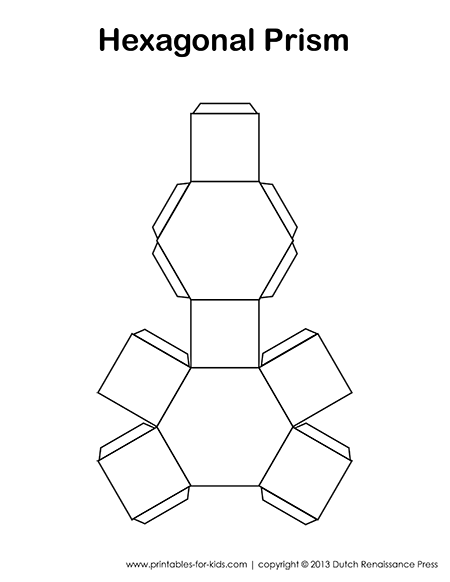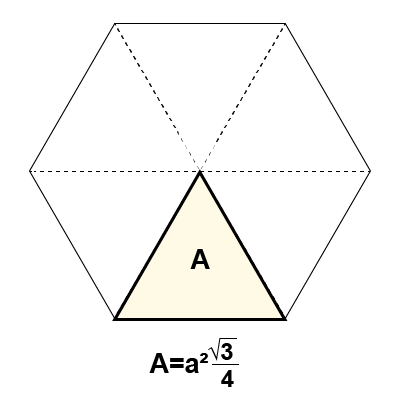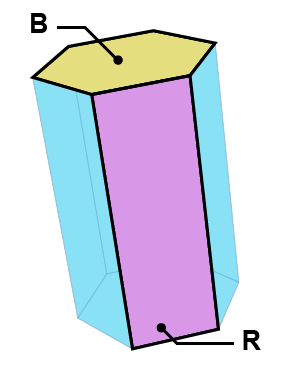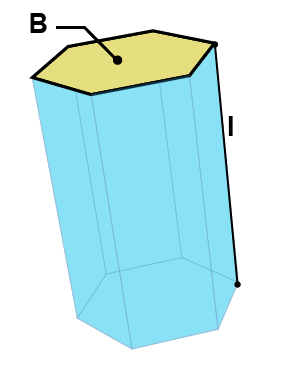# Hexagonal Prisms: Paper Models, Surface Area, Volume Formulas and Nets

Everything you ever wanted to know about hexagonal prisms. Whether you’re a student learning about polyhedrons for the first time, or a parent who needs a recap, by the time you get to the end of this webpage, you’ll be an expert.## Hexagonal Prism Definition

An hexagonal prism is a 3D object with two regular hexagonal caps and rectangular or square sides.Examples of Hexagonal Prisms

## Hexagonal Prism Paper Model and Net

Below is a free printable hexagonal prism net. Please open the pdf file by clicking on the image below. Then you will be able to download the file in your browser. (If you are unable to see the PDF, you may need an updated version of Adobe Acrobat Reader.)A printable hexagonal prism net pdf

[clearBoth]

## Construction Tutorial1. Print the Hexagonal Prism Net on some sturdy construction paper.2. Cut out the hexagonal prism net along its perimeter. Fold along the lines of the flaps and shapes that make up the net.3. Fold the pieces of the prism so that the hexagon caps are facing. Tape the flaps under the faces with scotch tape. Once all the flaps are taped, your hexagonal prism paper model is complete.
[clearBoth]

## Area of a Hexagon

You need to know how to calculate the area of a hexagon before you can calculate the surface area and volume of a hexagonal prism.A hexagon is made of 6 equilateral triangles.Area of a Triangle = 1/2 Base x Height.Formula for the Area of a Triangle.Formula for the Area of a Hexagon.

[clearBoth]

## Hexagonal Prism Surface Area FormulasHexagonal Prism Surface Area Formula:
6 R+ 2B
Where:
R = the Area of a Rectangle Face
B = the Area of an Hexagonal Face

The surface area of a prism is equal to the sum of the areas of its faces. An hexagonal prism is made up of 6rectangle faces and 2 hexagon faces.

If you happen to know R and B, then you’re all done. But what if you only know the length of a few sides of the hexagonal prism? No problem. Let’s take a look.Hexagonal Prism Surface Area Formula:
6al+ (3√3)a²
Where:
a = the length of an hexagon edge
l = the length of the hexagon prism

Notice that the first and second formulas are actually the same, since:

R =al

B=(3√3)a²/2

Instead of 6R +2B, we get: 6(al) + 2((3√3)a²/2) which is equal to the second formula: 6al+ (3√3)a²
[clearBoth]

## Hexagonal Prism Volume FormulasHexagonal Prism Volume Formula:
Volume =Bl
Where:
B = the area of a hexagon face
l = the length of the hexagon prism

Hexagonal Prism Volume Formula:
Volume = ((3√3)a²/2)l
Where:
a = the length of an hexagon edge
l = the length of the hexagon prism

Notice that the first and second formulas are actually the same, since:

B=(3√3)a²/2

Instead of Bl, we get: ((3√3)a²/2)l which is equal to the second formula: ((3√3)a²/2)l

## Vertices, Faces, and Edges

An hexagonal prism has 12 vertices, 8 faces, and 18 edges.